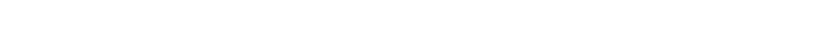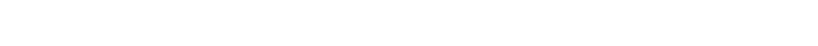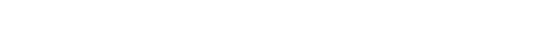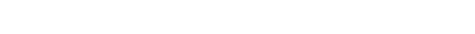### 简介

Spark正能应对这些问题。Spark是用Scala编写的，它提供了Scala、JAVA、Python和R的接口. PySpark一起工作的API。PySpark是用Python编写的Python API用来支持Spark的。PySpark以一种高效且易于理解的方式处理这一问题。因此，在本文中，我们将开始学习有关它的所有内容。我们将了解什幺是Spark，如何在你的机器上安装它，然后我们将深入研究不同的Spark组件。本文附有代码。

### 目录

1. Spark是什幺?

1. 在你的计算机上安装Apache Spark

1. 什幺是Spark应用程序?

1. 什幺是Spark会话?

1. Spark的分区

1. 惰性计算

1. Spark中的数据类型

### Spark是什幺?

Apache Spark是一个开源的分布式集群计算框架，用于快速处理、查询和分析大数据。### 在你的计算机上安装Apache Spark

#### 1. 下载Apache Spark

```tar xzvf spark-2.4.4-bin-hadoop2.7.tgz
sudo mv spark/ /usr/lib/```#### 2. 安装JAVA

```sudo apt install default-jre
sudo apt install openjdk-8-jdk```

#### 3.安装Scala构建工具(SBT)

SBT是Scala构建工具的缩写，它管理你的Spark项目以及你在代码中使用的库的依赖关系。

```echo "deb https://dl.bintray.com/sbt/debian /" | sudo tee -a /etc/apt/sources.list.d/sbt.list
curl -sL "https://keyserver.ubuntu.com/pks/lookup?op=get&search=0x2EE0EA64E40A89B84B2DF73499E82A75642AC823" | sudo apt-key add
sudo apt-get update
sudo apt-get install sbt```

#### 4. 配置SPARK

```cd /usr/lib/spark/conf/
cp spark-env.sh.template spark-env.sh
sudo gedit spark-env.sh```

```## 添加变量
JAVA_HOME=/usr/lib/jvm/java-8-openjdk-amd64
SPARK_WORKER_MEMORY=4g```

#### 5. 设置Spark环境变量

```## 打开bashrc
sudo gedit ~/bashrc```

```export JAVA_HOME=/usr/lib/jvm/java-8-openjdk-amd64
export SBT_HOME=/usr/share/sbt/bin/sbt-launch.jar
export SPARK_HOME=/usr/lib/spark
export PATH=\$PATH:\$JAVA_HOME/bin
export PATH=\$PATH:\$SBT_HOME/bin:\$SPARK_HOME/bin:\$SPARK_HOME/sbin
export PYSPARK_DRIVER_PYTHON=jupyter
export PYSPARK_DRIVER_PYTHON_OPTS='notebook'
export PYSPARK_PYTHON=python3
export PYTHONPATH=\$SPARK_HOME/python:\$PYTHONPATH```

`source ~/.bashrc`### 什幺是Spark应用程序?

Spark应用程序是Spark上下文的一个实例。它由一个驱动进程和一组执行程序进程组成。### 什幺是Spark会话?

Spark会话实例可以使用Spark在集群中执行用户自定义操作。在Scala和Python中，当你启动控制台时，Spark会话变量就是可用的:### Spark的分区

```from random import randint
# 创建一个随机数字的列表在10到1000之间
my_large_list = [randint(10,1000) for x in range(0,20000000)]
# 创建一个分区的列表
my_large_list_one_partition = sc.parallelize(my_large_list,numSlices=1)
# 检查分区数量
print(my_large_list_one_partition.getNumPartitions())
# >> 1
# 筛选数量大于等于200的数字
my_large_list_one_partition = my_large_list_one_partition.filter(lambda x : x >= 200)
# 在jupyter中运行代码
# 执行以下命令来计算时间
%%time
# 列表中元素的数量
print(my_large_list_one_partition.count())
# >> 16162207``````# 创建五个分区
my_large_list_with_five_partition = sc.parallelize(my_large_list, numSlices=5)
# 筛选数量大于等于200的数字
my_large_list_with_five_partition = my_large_list_with_five_partition.filter(lambda x : x >= 200)
%%time
# 列表中元素的数量
print(my_large_list_with_five_partition.count())
# >> 16162207```### 转换### 惰性计算

#### 现在，对于大型数据集，即使是一个基本的转换也需要执行数百万个操作。

```# 创建一个样本列表
my_list = [i for i in range(1,10000000)]
# 并行处理数据
rdd_0 = sc.parallelize(my_list,3)
rdd_0``````# 每个数增加4
rdd_1 = rdd_0.map(lambda x : x+4)
# RDD对象
print(rdd_1)
#获取RDD运算图
print(rdd_1.toDebugString())``````# 每个数增加20
rdd_2 = rdd_1.map(lambda x : x+20)
# RDD 对象
print(rdd_2)
#获取RDD运算图
print(rdd_2.toDebugString())``````# 创建一个文本文件的RDD，分区数量= 4
my_text_file = sc.textFile('tokens_spark.txt',minPartitions=4)
# RDD对象
print(my_text_file)
# 转换小写
my_text_file = my_text_file.map(lambda x : x.lower())
# 更新RDD对象
print(my_text_file)
print(my_text_file.toDebugString())``````# 分割单词
my_text_file = my_text_file.map(lambda x : x[:2])
# RDD对象
print(my_text_file)
print(my_text_file.toDebugString())
# 在所有的转换后得到第一个元素
print(my_text_file.first())````print(my_text_file.countApproxDistinct())`### Spark MLlib的数据类型

MLlib是Spark的可扩展机器学习库。它包括一些常用的机器学习算法，如回归、分类、降维，以及一些对数据执行基本统计操作的工具。

#### 局部向量

MLlib支持两种类型的本地向量:稠密和稀疏。当大多数数字为零时使用稀疏向量。要创建一个稀疏向量，你需要提供向量的长度——非零值的索引，这些值应该严格递增且非零值。

```from pyspark.mllib.linalg import Vectors
## 稠密向量
print(Vectors.dense([1,2,3,4,5,6,0]))
# >> DenseVector([1.0, 2.0, 3.0, 4.0, 5.0, 6.0, 0.0])
### 稠密向量
### Vectors.sparse( length, index_of_non_zero_values, non_zero_values)
### 索引应该严格递增且非零值
print(Vectors.sparse(10, [0,1,2,4,5], [1.0,5.0,3.0,5.0,7]))
# >> SparseVector(10, {0: 1.0, 1: 5.0, 2: 3.0, 4: 5.0, 5: 7.0})
print(Vectors.sparse(10, [0,1,2,4,5], [1.0,5.0,3.0,5.0,7]).toArray())
# >> array([1., 5., 3., 0., 5., 7., 0., 0., 0., 0.])```

#### 标签点

```from pyspark.mllib.regression import LabeledPoint
# 设置一个标签与一个稠密向量
point_1 = LabeledPoint(1,Vectors.dense([1,2,3,4,5]))
# 特征
print(point_1.features)
# 标签
print(point_1.label)```

#### 局部矩阵

```# 导入矩阵
from pyspark.mllib.linalg import Matrices
# 创建一个3行2列的稠密矩阵
matrix_1 = Matrices.dense(3, 2, [1,2,3,4,5,6])
print(matrix_1)
# >> DenseMatrix(3, 2, [1.0, 2.0, 3.0, 4.0, 5.0, 6.0], False)
print(matrix_1.toArray())
"""
>> array([[1., 4.],
[2., 5.],
[3., 6.]])
"""
# 创建一个稀疏矩阵
matrix_2 = Matrices.sparse(3, 3, [0, 1, 2, 3], [0, 0, 2], [9, 6, 8])
print(matrix_2)
# SparseMatrix(3, 3, [0, 1, 2, 3], [0, 0, 2], [9.0, 6.0, 8.0], False)
print(matrix_2.toArray())
"""
>> array([[9., 6., 0.],
[0., 0., 0.],
[0., 0., 8.]])
"""```

#### 分布式矩阵

```# 分布式数据类型——行矩阵
from pyspark.mllib.linalg.distributed import RowMatrix
# 创建RDD
rows = sc.parallelize([[1,2,3], [4,5,6], [7,8,9], [10,11,12]])
# 创建一个分布式行矩阵
row_matrix = RowMatrix(rows)

print(row_matrix)
# >> <pyspark.mllib.linalg.distributed.RowMatrix at 0x7f425884d7f0>
print(row_matrix.numRows())
# >> 4
print(row_matrix.numCols())
# >> 3```

```# 索引行矩阵
from pyspark.mllib.linalg.distributed import IndexedRow, IndexedRowMatrix
#创建RDD
indexed_rows = sc.parallelize([
IndexedRow(0, [0,1,2]),
IndexedRow(1, [1,2,3]),
IndexedRow(2, [3,4,5]),
IndexedRow(3, [4,2,3]),
IndexedRow(4, [2,2,5]),
IndexedRow(5, [4,5,5])
])
# 创建IndexedRowMatrix
indexed_rows_matrix = IndexedRowMatrix(indexed_rows)
print(indexed_rows_matrix.numRows())
# >> 6
print(indexed_rows_matrix.numCols())
# >> 3```

```from pyspark.mllib.linalg.distributed import CoordinateMatrix, MatrixEntry
# 用MatrixEntry创建
matrix_entries = sc.parallelize([MatrixEntry(0, 5, 2), MatrixEntry(1, 1, 1), MatrixEntry(1, 5, 4)])
# 创建坐标矩阵
c_matrix = CoordinateMatrix(matrix_entries)
# 列数
print(c_matrix.numCols())
# >> 6
# 行数
print(c_matrix.numRows())
# >> 2```

```# 导入库
from pyspark.mllib.linalg import Matrices
from pyspark.mllib.linalg.distributed import BlockMatrix
# 创建子矩阵块的RDD
blocks = sc.parallelize([((0, 0), Matrices.dense(3, 3, [1, 2, 1, 2, 1, 2, 1, 2, 1])),
((1, 1), Matrices.dense(3, 3, [3, 4, 5, 3, 4, 5, 3, 4, 5])),
((2, 0), Matrices.dense(3, 3, [1, 1, 1, 1, 1, 1, 1, 1, 1]))])
# 从子矩阵块的RDD中创建矩阵块，大小为3X3
b_matrix = BlockMatrix(blocks, 3, 3)
#每一块的列数
print(b_matrix.colsPerBlock)
# >> 3
#每一块的行数
print(b_matrix.rowsPerBlock)
# >> 3
# 把块矩阵转换为局部矩阵
local_mat = b_matrix.toLocalMatrix()
# 打印局部矩阵
print(local_mat.toArray())
"""
>> array([[1., 2., 1., 0., 0., 0.],
[2., 1., 2., 0., 0., 0.],
[1., 2., 1., 0., 0., 0.],
[0., 0., 0., 3., 3., 3.],
[0., 0., 0., 4., 4., 4.],
[0., 0., 0., 5., 5., 5.],
[1., 1., 1., 0., 0., 0.],
[1., 1., 1., 0., 0., 0.],
[1., 1., 1., 0., 0., 0.]])
"""```